Updated by josieslump on Oct 14, 2016
REPORT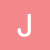josieslump
Owner
10 items   1 followers   0 votes   47 views

# Slope of a line-Thinglink Project

1

## How to Write a slope-intercept equation given an X-Y table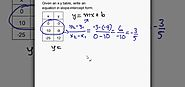This website helps focuses on writing the slope from a given X-Y table. This resource is helpful to my thinklink because it perfectly fits with my focus area-slope of a line. It is also easy to navigate and gives great examples.

2

## Finding the Slope of a Line from Two Points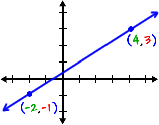This website focuses on finding slope given two different point coordinates. I chose this resource because it fits perfectly with my focus area-finding slope. It is also very clear in its explanation of finding slope, is easy to use, and gives great visual examples.

3

## What is Delta? - Definition & Concept - Video & Lesson Transcript | Study.comThis video explains what is meant by the Greek sign delta. I chose this source because the formula for slope uses the Greek sign delta. This explanation helps students understand why we use this symbol and what it means. It is clear in its explanation.

4

## Proportional Relationships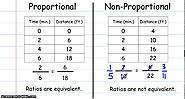This picture focuses on proportional relationships. I chose this source because proportional relationships means they have the same slope, and finding slope is my focus. This picture makes very clear the difference between proportional and unproportional relationships, which is why I chose it.

5

## 'Find the slope of a graph'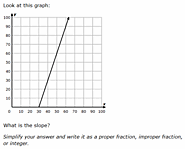This source is an interactive way for students to practice their skills in finding slope. I chose this source because it directly asks the students to do what my focus is-finding slope. It is a simple quiz tool that just asks the students for their answer. This is a good assessment tool once students have learned how to find slope.

6

## Proving slope is constantThis video is a great application of finding slope: proving similar triangles. It shows how students can use slope to prove that triangles are similar. I chose this source because it directly applies my focus of finding slope to other areas of math. The video does a great job giving a clear explanation that students can understand.

7

## Practice Quiz

This website is an online quiz. It quizzes students over finding slope. I chose this source because it directly relates to finding slope and is a great tool for assessing the student' s knowledge of the content. It is easy to use and clear in its directions.

8

## How to find the slope of a lineThis is a video explanation of how to find the slope of a line given two points or its graph whether the slope is positive, negative or undefined or the line is vertical or horizontal.I chose this source because it clearly explains how to find the slope of the line and gives great examples. The video is fun and a different teaching method than straight lecture.

9

## Formula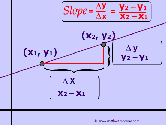This source is a picture and visual representation of the definition of slope of a line. I chose this source because it gives both the formula definition and a visual representation of what the formula means. It explains the use of delta and what it looks like graphically.

10

## Slopes of Triangles worksheet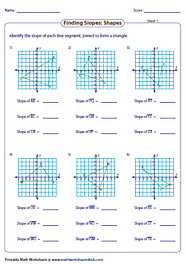This source is a worksheet for students in finding the slope in triangles. I chose this source because it directly applies what we are learning about slopes to something other than lines. I think this worksheet is clear in its directions and uses good examples. This worksheet would be a good way to assess the child's knowledge and understanding of the concept.